# RS Aggarwal Class 10 Solutions Chapter 9 - Mean Median Mode Ex 9D(9.4)

## RS Aggarwal Class 10 Chapter 9 - Mean Median Mode Ex 9D(9.4) Solutions Free PDF

The solutions of Class 10 for Chapter 9 lets you practice different questions on a regular basis and is the key to scoring good marks in the exam. The RS Aggarwal Solutions Class 10 Chapter 9 Mean Median Mode Ex 9D is the best study material that you can refer to while preparing for your Class 10 exam. All the solutions mentioned below are solved in a step-by-step way which helps you to understand the logic behind such questions easily.

These solutions can be referred by students whenever they have any doubt or get stuck while solving any particular question. To excel in the exams, you should practice the RS Aggarwal Class 10 Solutions for Maths regularly. Firstly, practice the difficult topics to clear your concepts and develop your problem-solving skills. It will help you solve questions in quick-time and score good marks.

## Download PDF of RS Aggarwal Class 10 Solutions Chapter 9– Mean Median Mode Ex 9D (9.4)

Q1: Find the median of the following data by making a ‘less than give’

 Marks 0-10 10-20 20-30 30-40 40-50 50-60 60-70 70-80 80-90 90-100 No. of students 5 3 4 3 3 4 7 9 7 8

Sol:

The frequency distribution table of less than type is given as follows:

 Maks (upper class limits) Cumulative frequency (cf) Less than 10 5 Less than 20 5+3=8 Less than 30 8+4=12 Less than 40 12+3=15 Less than 50 15+3 = 18 Less than 60 18+4 = 22 Less than 70 22+7 =29 Less than 80 29+9 =38 Less than 90 38+7 =45 Less than 100 45+8 =53

Taking upper class limits of class intervals on x-axis and their respective frequencies on y-axis, its ogive can be drawn as follows: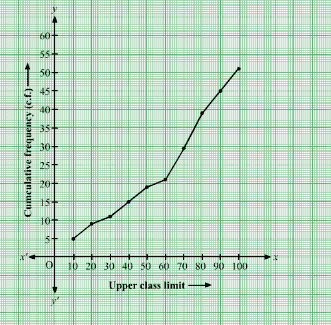Here, N=53,

$\Rightarrow \frac{N}{2}= 26.5$

Mark the point A whose ordinate is 26.5 and its x-coordinate is 66.4.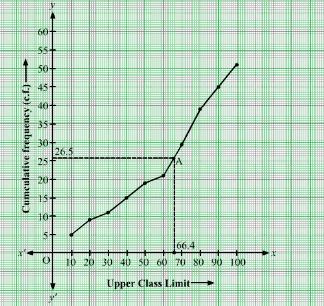The median of the data is 66.4.

Q2: The given distribution shows the number of wickets taken by the bowlers in one-day international cricket matches:

 No. of wickets Less than 15 Less than 30 Less than 45 Less than 60 Less than 75 Less than 90 Less than 105 Less than 120 No. of bowlers 2 5 9 17 39 54 70 80

Sol:

Taking upper class limits of class intervals on x-axis and their respective frequencies on y-axis, its ogive can be drawn as follows: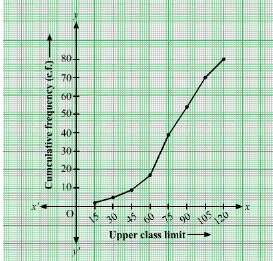Here, N=80,

$\Rightarrow \frac{N}{2}= 40$

Mark the point A whose ordinate is 40 and its x-coordinate is 76.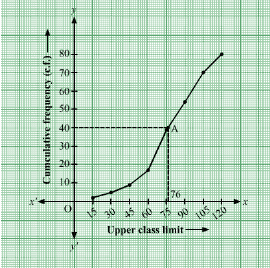The median of the data is 76.

Q3: Draw a ‘more than’ ogive for the data given below which gives the marks of 100 students.

 Marks 0-10 10-20 20-30 30-40 40-50 50-60 60-70 70-80 No. of students 4 6 10 10 25 22 18 5

Sol:

Taking upper class limits of class intervals on x-axis and their respective frequencies on y-axis, its ogive can be drawn as follows:

 Marks (lower class limits) Cumulative frequency (cf) More than 0 96+4 = 100 More than 10 90+6 = 96 More than 20 80+10=90 More than 30 70+10=80 More than 40 45+25=70 More than 50 23+22=45 More than 60 18+5=23 More than 70 5

Taking the lower class limits on x-axis and their respective cumulative frequencies on y-axis, its ogive can be obtained as follows: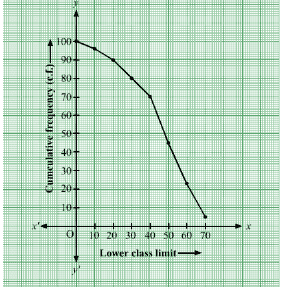Q4: The height of 50 girls of class X of a school are recorded as follows:

 Height ( in cm) 135-140 140-145 145-150 150-155 155-160 160-165 No. of girls 5 8 9 12 14 2

Draw a ‘more than type’ ogive for the above data.

Sol:

The frequency distribution table of more than type is as follows:

 Height (in cm) (lower class limits) Cumulative frequency (cf) More than 135 45+5=50 More than 140 37+8=45 More than 145 28+9=37 More than 150 16+12=28 More than 155 14+2=16 More than 160 2

Taking the lower class limits on x-axis and their respective cumulative frequencies on y-axis, its ogive can be obtained as follows: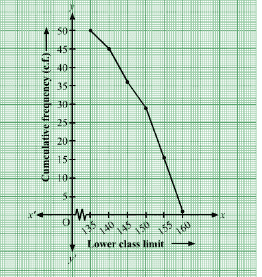Q5: The monthly consumption of electricity ( in units) of some families of a locality is given in the following frequency distribution:

 Monthly consumption (in units) 140-160 160-180 180-200 200-220 220-240 240-260 260-280 No. of families 3 8 15 40 50 30 10

Sol:

The frequency distribution table of more than type is as follows:

 Height (in cm) (lower class limits) Cumulative frequency (cf) More than 140 3+153=156 More than 160 8+145=153 More than 180 15+130=145 More than 200 40+90=130 More than 220 50+40=90 More than 240 30+10=40 More than 260 10

Taking the lower class limits on x-axis and their respective cumulative frequencies on y-axis, its ogive can be obtained as follows: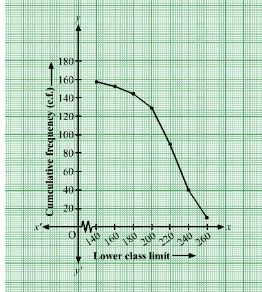Q6: The following table gives the production yield per hectare of wheat of 100 farms of a village.

 Production yield (kg/ha) 50-55 55-60 60-65 65-70 70-75 75-80 No. of farms 2 8 12 24 38 16

Change the distribution to ‘a more than type’ distribution and draw its ogive. Using ogive, find the median of the given data.

Sol:

The frequency distribution table of more than type is as follows:

 Production yield (kg/ha)(lower class limits) Cumulative frequency (cf) More than 50 2+98=100 More than 55 8+90=98 More than 60 12+78=90 More than 65 24+54=78 More than 70 38+16=54 More than 75 16

Taking the lower class limits on x-axis and their respective cumulative frequencies on y-axis, its ogive can be obtained as follows: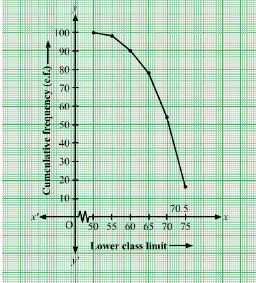Here, N=100,

$\Rightarrow \frac{N}{2}= 50$

Mark the point A whose ordinate is 50 and its x-coordinate is 70.5.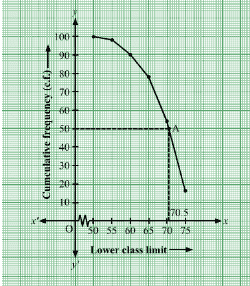The median of the data is 70.5

Q7: The table below shows the weekly expenditures on food of some household in a locality:

 Weekly introduction (in Rs.) No. of household 100-200 5 200-300 6 300-400 11 400-500 13 500-600 5 600-700 4 700-800 3 800-900 2

Draw a ‘less than type ogive’ and a ‘more than type ogive’ for this distribution.

Sol:

The frequency distribution table of less than type is as follows:

 Weekly expenditure ( in Rs) (Upper class limits) Cumulative frequency (cf) Less than 200 5 Less than 300 5+6=11 Less than 400 11+11=22 Less than 500 22+13=35 Less than 600 35+5=40 Less than 700 40+4=44 Less than 800 44+3=47 Less than 900 47+2=49

Taking the lower class limits on x-axis and their respective cumulative frequencies on y-axis, its ogive can be obtained as follows: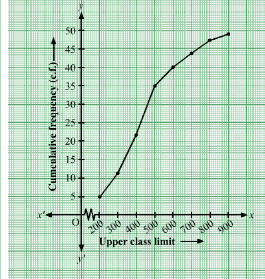Now,

The frequency distribution table of more than type is as follows:

 Weekly expenditure (in Rs) ( lower class limits) Cumulative frequencies (cf) More than 100 44+5=49 More than 200 38+6=44 More than 300 27+11=38 More than 400 14+13=27 More than 500 9+5=14 More than 600 5+4=9 More than 700 2+3=5 More than 800 2

Taking the lower class limits on x-axis and their respective cumulative frequencies on y-axis, its ogive can be obtained as follows: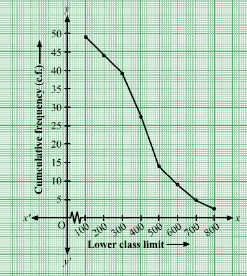Q8: From the following frequency distribution , prepare the ‘more than ogive’.

 Score No. of candidates 400-450 20 450-500 35 500-550 40 550-600 32 600-650 24 650-700 27 700-750 18 750-800 34 Total 230

Also find the median.

Sol:

From the given table, we may prepare the ‘more than’ frequency table as shown below:

 Score Number of candidates More than 750 34 More than 700 52 More than 650 79 More than 600 103 More than 550 135 More than 500 175 More than 450 210 More than 400 230

We plot the points A(750,34), B(700,50), C(650,79), D(600,103), E(550,135), F(500,175), G(450,210) and H(400,230).

Join AB,BC,CD,DE,EF,FG,GH and HA with a free hand to get the curve representing the ‘more than types’ series.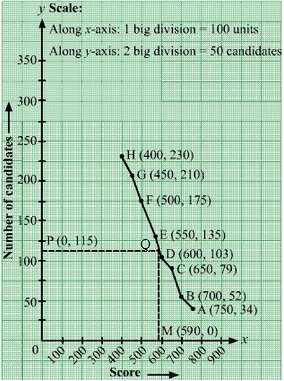Here N= 230

$\Rightarrow \frac{N}{2}= 115$

From P(0,115), draw PQ meeting the curve at Q.

Draw QM meeting at M.

Clearly, QM = 590 units

Hence, median = 590 units

Q9: The marks obtained by 100 students of a class in an examination are given below:

 Marks No. of students 0-5 2 5-10 5 10-15 6 15-20 8 20-25 10 25-30 25 30-35 20 35-40 18 40-45 4 45-50 2

Draw cumulative frequency curves by using

(i) ‘Less than’ series and

(ii) ‘More than’ series

Hence find the median.

Sol:

(i) From the given table, we may prepare the ‘less than’ frequency table as shown below:

 Marks No. of students Less than 5 2 Less than 10 7 Less than 15 13 Less than 20 21 Less than 25 31 Less than 30 56 Less than 35 76 Less than 40 94 Less than 45 98 Less than 50 100

We plot the points A(5,2), B(10,7), C(15,13), D(20,21), E(25,31), F(30,56), G(35,76), H(40,94), I(45,98), and J(50,100).

Join AB,BC,CD,DE,EF,FG,GH,HI,IJ and JA with a free hand to get the curve representing the ‘less than types’ series.

(ii) More than series:

 Marks No. of students More than 0 100 More than 5 98 More than 10 93 More than 15 87 More than 20 79 More than 25 69 More than 30 44 More than 35 24 More than 40 6 More than 45 2

Now on the same graph paper, we plot the points (0,100), (5,98), (10,94), (15,76), (20,56), (25,31), (30,21), (35,13), (40,6) and (45,2).

Join, with a free hand to get the ‘more than type’ series.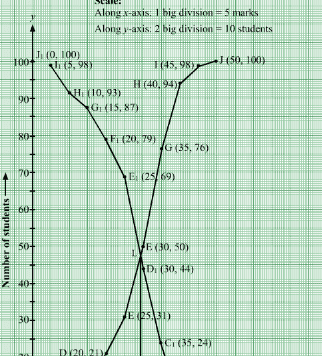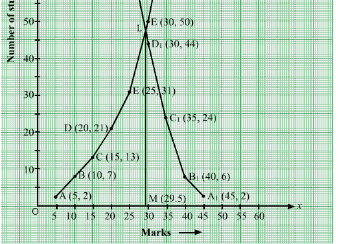The two curves intersect at point L. Draw $LM \perp OX$ cutting the x-axis at M. Clearly, M= 29.5.

Hence, Median = 29.5

Q10: From the following data, draw two types of cumulative frequency curves and determine the median

 Height ( in cm) Frequency 140-144 3 144-148 9 148-152 24 152-156 31 156-160 42 160-164 64 164-168 75 168-172 82 172-176 86 176-180 34

Sol:

(i) Less than series:

 Marks No. of students Less than 144 3 Less than 148 12 Less than 152 36 Less than 156 67 Less than 160 109 Less than 164 173 Less than 168 248 Less than 172 330 Less than 176 416 Less than 180 450

We plot the points A(144,3), B(148,12), C(152,36), D(156,67), E(160,109), F(164,173), G(168,248), H(172,330), I(176,416), and J(180,450).

Join AB,BC,CD,DE,EF,FG,GH,HI,IJ and JA with a free hand to get the curve representing the ‘less than types’ series.

(ii) More than series:

 Marks No. of students More than 140 450 More than 144 447 More than 148 438 More than 152 414 More than 156 383 More than 160 341 More than 164 277 More than 168 202 More than 172 120 More than 176 34

Now on the same graph paper, we plot the points  $A_{1}(140,4510) ,B_{1}(144,447), C_{1}(148,447), D_{1}(152,414), E_{1}(156,383), F_{1}(160,341),G_{1}(164,277), H_{1}(168,202),I_{1}(172,120) and J_{1}(176,34)$

Join, $A_{1}B_{1}, B_{1}C_{1}, C_{1}D_{1},D_{1}E_{1},E_{1}F_{1},F_{1}G_{1},G_{1}H_{1},H_{1}I_{1},I_{1}J_{1}$ with a free hand to get ‘more than type’ series.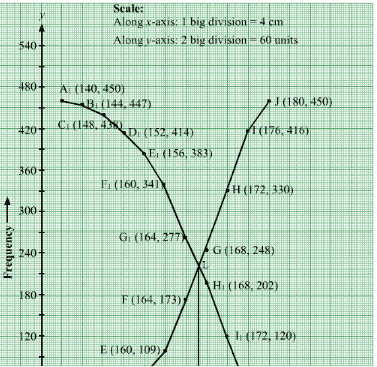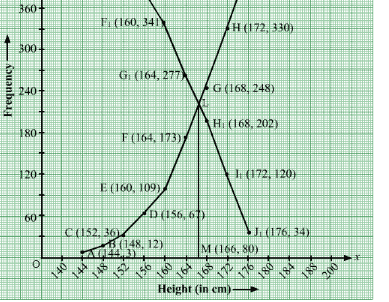The two curves intersect at point L. Draw $LM \perp OX$ cutting x-axis at M. Clearly, M= 166 cm.

Hence, Median = 166 cm.

### Key Features of RS Aggarwal Class 10 Solutions Chapter 9 – Mean Median Mode Ex 9D (9.4)

• It will improve your speed and accuracy along with overall academic performance.
• RS Aggarwal Maths Solutions helps in practicing different types of questions.
• It gives an idea to solve difficult and tricky questions in a simple way.
• These solutions are based on the recent syllabus of the CBSE Class 10.

#### Practise This Question

Above picture is a bi-focal lens, used to correct a presbyopic person, what is region 1 and 2?